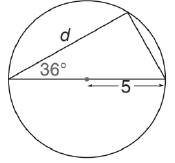Chapter 11.3, Problem 19EElementary Geometry For College St...

7th Edition
Alexander + 2 others
ISBN: 9781337614085

Solutions

Chapter
SectionElementary Geometry For College St...

7th Edition
Alexander + 2 others
ISBN: 9781337614085
Textbook Problem

In Exercises 15 to 20, use the sine, cosine, or tangent ratio to find the lengths of the indicated sides to the nearest tenth of a unit.To determine

To find:

The lengths of the indicated sides to the nearest tenth of a unit using the sine, cosine or tangent ratio.

Explanation

Given:

The triangle inscribed in the circle with the indicated sides is shown below.

Definition used:

The trigonometric ratios:

Calculation:

Let α=36.

From the figure we see that the radius of the circle is 5.

Therefore the diameter is 25=10.

We know that the angle inscribed in a semicircle is a right angle.

Hence the hypotenuse of the triangle is the diameter of the circle, whose value is 10.

We need to find the value of d.

cos36=d10

Let us use Ti-83 calculator to find the value of cos36.

Press ON in Ti-83 calculator.

Since we need to find the value of degree, we need to change the mode to degrees

Still sussing out bartleby?

Check out a sample textbook solution.

See a sample solution

The Solution to Your Study Problems

Bartleby provides explanations to thousands of textbook problems written by our experts, many with advanced degrees!

Get Started

Given P=I2R,I=4, and R=2000, find P.

Elementary Technical Mathematics

Factor each expression in Problems 7-18 as a product of binomials. 11.

Mathematical Applications for the Management, Life, and Social Sciences

Expand each expression in Exercises 122. (y1y)2

Finite Mathematics and Applied Calculus (MindTap Course List)

In Exercises 4562, find the values of x that satisfy the inequality (inequalities). 57. (2x 3)(x 1) 0

Applied Calculus for the Managerial, Life, and Social Sciences: A Brief Approach

True or False: 23xdx is an improper integral.

Study Guide for Stewart's Single Variable Calculus: Early Transcendentals, 8th